## A puck of mass m moving at speed v on a horizontal, frictionless surface is stopped in a distance d because a hockey stick exerts on an oppo

Question

A puck of mass m moving at speed v on a horizontal, frictionless surface is stopped in a distance d because a hockey stick exerts on an opposing force of magnitude F on it.

F = (mv^2) / 2d

If the stopping distance d increases 40%, by what percent does the average force needed to stop the puck change, assuming that m and v are unchanged?

(Fnew – F) / F = ? %

in progress 0
2 weeks 2021-08-31T23:10:48+00:00 1 Answers 0 views 0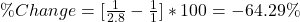Explanation:

Assuming that m and v are unchanged.

For this case we have the following formula for the force: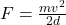For this case the new force would be given: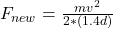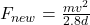And for this case we can calculate the % like this: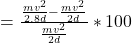And doing the algebra we got: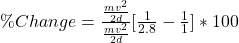So then the force decrease 64.29 percent respect the original force.